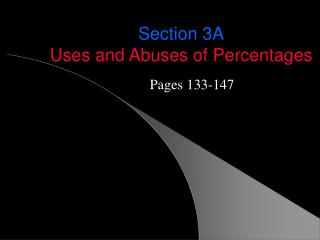DownloadDownload PresentationSection 3A Uses and Abuses of Percentages

# Section 3A Uses and Abuses of Percentages

Télécharger la présentation## Section 3A Uses and Abuses of Percentages

- - - - - - - - - - - - - - - - - - - - - - - - - - - E N D - - - - - - - - - - - - - - - - - - - - - - - - - - -
##### Presentation Transcript

1. Section 3AUses and Abuses of Percentages Pages 133-147

2. fraction decimal percentage 3-A per cent = “per 100” = “divided by 100” 47% = 47/100 = .47 2% = 2/100 = .02 813% = 813/100 = 8.13

3. 3-A To convert a percentage to a decimal simply divide by 100 or move the decimal two places to the left 45% = 45/100 = .45 1.2% = 1.2/100 = .012 To convert a decimal to a percentage simply multiply by 100% (=1) or move the decimal two places to the right: .16 = .16 * 100% = 16% 2.3 = 2.3 * 100% = 230%

4. 3-A To convert a percentage to a fraction simply divide by 100 and reduce as necessary: 45% = 45/100 = 9/20 1.2% = 1.2/100 = 12/1000 = 3/250 To convert a fraction to a percentage simply divide to get a decimal and then move decimal two spaces to the right: 1/5 = .20 = .20 * 100% = 20% 5/4 = 1.25 = 1.25*100% = 125%

5. 3-A 3 Ways of Using Percentages • As fractions – “Percent of” • To describe change over time • For comparison

6. 3-A 1. Percent “of “ - (multiply) ex1/134 A newspaper reports that 44% of 1069 people surveyed said that the president was doing a good job. How many people said this? 44% * 1069 = .44*1069 = 470.36 = 470 people

7. 3-A More Practice 37/148 A is 75 and B is 480. A is ____________ percent of B. B is ____________ percent of A. 46/148345 blooming tulips in a field of 398 tulips. The blooming tulips are _______ percent of the field.

8. 3-A 2. Percents are often used to describe how a quantity changes over time Given: original value and new value absolute change = new value – original value relative change

9. 3-A Absolute Change vs. Relative Change 51/148 John’s salary grew from \$20000 in 2000 to \$28000 in 2003. absolute change=\$28000 – \$20000 = \$8000 relative change = \$8000/\$20000 = .40 = 40% John’s salary in 2003 was 40% more than his salary in 2000 [John’s salary in 2003 was 28000/20000 = 1.40 =140% ofhis salary in 2000.]

10. 3-A Absolute Change vs. Relative Change ex4/137A computer you bought three years ago for \$1000 is today worth only \$300. absolute change=\$300 – \$1000 = -\$700 relative change = -\$700/\$1000 = -.70 = -70% The value of the computer today is 70% less than its value three years ago. [The computer’s value today is 300/1000 = .30 =30% ofthe value in 2003.]

11. 3-A Absolute Change vs. Relative Change ex2/136Today,my stock doubled in price from \$10 to \$20. absolute change=\$20 – \$10 = \$10 relative change = \$10/\$10 = 1 = 100% The value of stock today is 100% more than yesterday’s value. [The stock’s value today is 20/10 = 2 =200% ofthe value yesterday.] What if my stock tripled in value?

12. 3-A Percentage Of vs. More (or Less) Than(page 140) If the new value is P% more than the original value, then the new value is (100+P)%of the original value. If the new value is P% less than the original value, then the new value is (100-P)%of the original value.

13. 3-A 3. Percents are often used to compare two values. Given:comparedvalue andreferencevalue absolute difference = compared value  ‘reference’ value

14. 3-A 57/149 The daily circulation of the Wall Street Journal is ≈1.77 million. The daily circulation of the New York Times is ≈ 1.07 million [Find the absolute and relative difference. Assume that the first quantity is the compared value and the second is the reference value.] Absolute difference = 1770000-1070000=700000 The WSJ has 700000 more readers than the NYT. Relative difference = 700000/1070000 = .654 = 65.4% The WSJ has 65.4% more readers than the NYT.

15. 3-A Solving Percentage Problems 77/150 You purchase a bicycle with a labeled (pre-tax) price of \$699. The local sales tax rate is 7.6%. What is your final cost? 78/150 The final cost of your new shoes is \$107.69. The local sales tax rate is 6.2%. What was the labeled (pre-tax) price. 81/150 Your dinner bill is \$18.75. You leave \$22. What percent tip did you leave?

16. 3-A Homework Pages 147-150 # 50, 55, 56, 58, 61, 79, 80, 82Express your answers in sentence form.